# Distributed Random Access Scheduling

We consider a network of wireless routers. The routers that are close together can interfere if they transmit simultaneously. So schedules need to avoid such collisions. We want each each wireless node to achieve a transmission rates that equals its arrival rate. One might want to implement a policy like MaxWeight or simply estimate the vector of arrival rates and accordingly choose the correct transmission rate. However, this is complicated by the fact that the routers do not know the arrival and transmission rates of their neighbors; all they can do is sense if their neighbors are transmitting or not.

We outline a clever and surprising result that shows that despite these limitations, there are algorithms that a stabilizing throughput is achievable for the maximum possible set of arrival rates. The model is as follows

• We consider a graph$G=({\mathcal J},{\mathcal E})$, where${\mathcal J}$ indexes a set of nodes and each edge$(i,j)\in{\mathcal E}$ indicates that the nodes$i$ and$j$ cannot transmit simultaneously. Thus the set of admissible schedules is given by the setNote the schedules are the independent sets of the graph$G$.
• As we saw with MaxWeight, the maximal region the node sizes can be stabilized for the set of arrival rates in the convex hull of${\mathcal S}$, which we denote by$<{\mathcal S}>$.

Now, parameterized by$r=(r_j:j\in{\mathcal J})$, we consider a Markov chain model of this system.

• With each node$j\in{\mathcal J}$ we associate a rate$R_i=e^{r_i}$. If$j$ is not transmitting all its neightbours are not sending (i.e.$\sigma_j=0$ and$\sigma_i =0$, for all$(i,j)\in{\mathcal E}$) then after an exponential distributed time with rate$R_i$ the node will start to transmit.
• If$j$ is transmitting, then after an exponentially distributed time of rate 1, the node will stop transmitting.

Notice the Markov chain above does not consider the queueing dynamics of the system, it is just interested in the states of the nodes, whether they are sending or not.

The following is a fairly straight-forward calculation for our Markov chain

Proposition: The stationary distribution,$\pi^r$, of the Markov chain just described has the form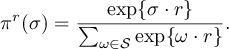for$\sigma\in{\mathcal S}$. Hence, the throughput a node$j$ under this stationary distribution isThe process is reversible and so above proposition is a straight-forward check of the detailed balance equations.

We now study the following function of$r$ and$\lambda$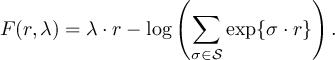This intuition behind$F(r,\lambda)$ is as follows. If we want to achieve a rate$\lambda\in <{\mathcal S}>$ then, since it belongs to the convex hull of${\mathcal S}$, there exist a probability distribution$\nu$ such that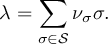The function$-F$ is upto a constant the relative entropy between$\nu$ and$\pi^r$ (which measures the distance between these two distributions):Here$H(\nu)$, the entropy of$\nu$, is a constant in$\pi$.

The relative entropy is minimized when$\nu$ is equal to$\pi^r$, so as a result we want to choose parameters$r$ to solve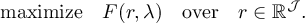The following proposition helps achieve this task:

Proposition: The function$F$ has with derivativeMoreover$F(r,\lambda)$ is a strictly concave function of$r$ for$\lambda\in<{\mathcal S}>^\circ$ and is maximize at$r^*$ satisfying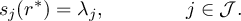Proof: Notice thatTo confirm the strict concavity of$F$ we analyse its Hessian. Observewhere${\mathbf \sigma}=({\mathbf \sigma}_j : j\in{\mathcal J})$ is a random variable with distribution$\pi^r$. Thus the Hessian of$F$ is$H_F= -{\mathbb E}_{\pi^r} [ ({\mathbf \sigma} - \mu)({\mathbf \sigma} - \mu)^{T} ]$, where$\mu:= ({\mathbb E}_{\pi^r}[{\mathbf \sigma}_j] : j\in{\mathcal J} )$, so concavity is immedidiate from negative semi-definiateness of the Hessian. If it were not negative definate we would have$0= -\eta^T H_F \eta = {\mathbb E}_{\pi^r} [ \eta^{T} ({\mathbf \sigma} - \mu)({\mathbf \sigma} - \mu)^{T}\eta ]$, thus$\eta^{T} ({\mathbf \sigma} - \mu) =0$ with probability one. Since for each unit vector$e_i$ the event${\mathbf \sigma}=e_i$ occus with positive probability, so,$\eta^{T} (e_i - \mu) =0$. In otherwords,$\eta_i= \eta^T \mu = const$ for all$i\in{\mathcal J}$. But${\mathbf \sigma}=0$ occurs also, so$\eta^{T} (0- \mu) =0$. So these last two observations imply$\eta_i =0$ for all$i\in{\mathcal J}$ and thus,$H_F$ is negative definate.

By differentiability if there is an optimum then it must satisfy the condition$s_i(r)= \lambda_i$, for all$i\in{\mathcal J}$. Note that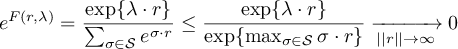The above limit shows that the optimum must be achieved finitely, since solutions diverge away from zero. The limit to zero above holds (uniformly) as$|| r ||\rightarrow\infty$ since for an open ball around$\lambda$,$B(\lambda,\epsilon)\subset <{\mathcal S}>$ for some$\epsilon>0$, so$\lambda\cdot r + \epsilon ||r||_1 \leq \max_{\sigma\in{\mathcal S}} \sigma\cdot r$$\square$

If we want to determine rates$r$ so that the output distribution of our network$s(r)$ equals the input rate$\lambda$ then we we should try optimize$F(r,\lambda)$. In particular a gradient decent algorithm will achieve this taskThe most most important observation is that the parameters$\lambda_i$ and$s_i(r)$ are estimatable from local information at each node$i$. I.e., node$i$ does need to know the load$\lambda_i$ or the parameter$r_j$ at other nodes inorder to observe$\lambda_i$ and$s_i(r(k))$ yet still it can reach a rate .

This gives the main idea of this line of work. However, there are many details required to deal with the recurssion . First,$\lambda_i$ and$s_i(r(k))$ must be estimated and inparticular$s_i(r(k))$ should be a good estimate of the stationary distribution of the Markov process with$r$ fixed. This involves taking increasingly time steps between interations in$k$ whose length are determined by the mixing time of the Markov chain towards$\pi^r$. Second, we donât consider queueing dynamics on top of these access rates. We might want to prove stability of the corresponding queueing process. Third, we might be interested in adapting the algorithm towards a different optimal choice of$\lambda$. This is also possible with some tweaking of the the algorithm.